# Astrophysics

Astrophysics is a hybrid of Physics and Astronomy that attempts to explain the physical workings of the celestial objects and phenomena. Astrophysics has two subdivisions: theoretical, and applied or experimental.

## Research focus

Research in astrophysics addresses a great variety of topics.

### Stars

• formation and evolution
• magnetic field properties
• stellar convection

### Stellar atmospheres

• nature of spectral analysis
• electron density

### The Galaxy (the Milky Way)

• interstellar gas and dust

### Galaxies

• formation and evolution
• modeling the environs of galactic-centre massive black holes;
• the structure and dynamics of galactic bars;
• the flow of gas into and out of galaxies;
• the role of active galactic nuclei in limiting the growth of galaxies.

### Astroparticles

Astroparticles are particles that are found in the universe. The study of astroparticles, astroparticle physics, is a multidisciplinary field that involves particle physics, astronomy, astrophysics and cosmology. Particle physics studies the nature of the structure of matter and fundamental laws governing their interactions, basically astroparticle physics studies the smallest aspects of the universe. Astronomy and astrophysics study the universe on a larger scale from its origin and evolution since the Big Bang. Cosmology in turn links the theories of particle physics with theories of the Universe at its earliest moments. 

Astroparticle studies employ different types of facilities and appliances. Underground laboratories to study neutrinos and dark matter are used to shield experiments from the background of cosmic muons. Land based observatories (telescopes and antennas) study very high energy gamma rays, neutrinos and very high energy cosmic rays. Satellite observatories are used to study high energy gamma rays, cosmic rays and gravitational waves.

#### Neutrinos

Neutrinos are the second most abundant particles in the universe. Neutrinos are produced in nuclear reactions occurring within stars. Neutrinos can also be artificially produced in high-energy collisions. Neutrinos bombard the earth constatantly in vast numbers. Each square centimetre of the human body is traversed by 60 billion neutrinos per second.

Neutrinos are not charged particles, so they don’t interact with matter through the electromagnetic force. They do not even interact through the strong force that holds together the nucleus of an atom. Neutrinos can only be affected by other particles through the weak force (e.g. in the nuclear fusion in the stars). Because they do not react readily, they can pass through almost any kind and thickness of matter without leaving any traces and with no deviation in their direction of motion, a direction they have had had since the instant of their origin.

There are three types or flavours of neutrinos: electronic, muonic or tauonic. A neutrino can oscillate from one flavour into another over long distances. 

Flavour oscillation may demonstrate that neutrinos do in fact have mass. Given their abundance, it is possible that neutrinos may have been invovled in some types of symmetry changes or breaks, such as the matter-antimatter ratio, believed to be very close in the early stages of the universe. This ratio of matter and anti-matter is now very asymmetrical; matter has an overwhelmingly higher ratio to antimatter. 

### Black holes

(See scholarpedia.)

### Astrochemistry

Astrochemistry involves the study of the role of the chemical bond and organic chemistry in nature on a cosmic scale. Over 140 molecules have been identified in the interstellar gas and circumstellar shells. To date the largest is a carbon chain with 13 atoms and a molecular weight of 147.

### Curvature

The curvature of space, or spacetime geometry, also refers to the shape of the universe. The concept of curved space originated in the work of Albert Einstein’s Theory of General Relativity. Space is united with time in a geometric spacetime which can be curved or flat. It may be positively or negatively curved or its curvature may be zero.

Positive curvature (often depicted graphically as a spherical model) is called a closed universe: Spacetime expands from a volume of zero, reaches the maximum point of expansion and the contracts to zero volume in the Big Crunch.

Negative spacetime geometry is depicted as a pseudosphere, for example a hyperboloid which appears to curve outward from a constricted middle, like two cones end to end or a double ended funnel such as might be typical of an hour-glass shape. Negative curvature spacetime has infinite volume and expands forever.

Zero curvature is a flat space and extends infinitely in any directions; it too, is an open universe that expands forever.

Einstein employed classic physics (not quantum physics) to describe the large scale of the universe. His general theory of relativity basically connotes curvature in spacetime in a given direction. That direction is determined by the energy and momentum of everything in the spacetime, i.e. not spacetime itself. He was saying, in other words, that gravity and non-gravity, geometry and non-geometry were combined. So, all types of matter and energy, everything that is involved in every type of force, exist in spacetime and also influence the shape of the spacetime.

There are some basic assumptions about the nature of the geometry of spacetime. One assumption is that space and time can be separated (though the extreme nature of such phenomena as a black hole may be an exception) and space time can be perceived as space changing with time. Another assumption in the spacetime model is that there is maximal symmetry: space is isotropic—looks the same in every direction; and space is homogenous—looks the same at every point. Curvature has to be the same at every point.

Theoretically, there are three types of energy that effect the geometry of space time:

1. Vacuum energy;
3. Matter.

One possible explanation of what determines the curvature of space time, whether the universe is open or closed, is the total energy density (designated$r\,$) of the universe. Vacuum energy theoretically leads to a constant energy density referred to as the cosmological constant. Energy density from radiation and matter of the universe may lead to an open, closed of flat universe.The three density ratios (Ω omega) and the corresponding cosmological morphology. (Goddard Space Flight Center WMAP Cosmology)

If the universe is closed, then it has positive curvature and the total energy density has to be greater than the energy needed for a flat universe. Density for Zero curvature, flat space, is called, critical density$r_0\,$

Using a simple formula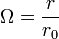$\Omega=\frac{r}{r_0}$
(or in some notations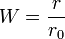$W=\frac{r}{r_0}$ where W is the critical density)

In a closed universe$\Omega>1\,$

In an open universe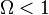$\Omega<1\,$

In a flat universe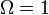$\Omega=1\,$

### Dark matter

In the past few decades there has been mounting evidence that there is not enough matter in the universe to account for the amount of gravity needed to hold galaxies together. This has led to the idea that there is dark matter, matter that can not be seen in the normal way, in any known spectrum. And there seems to be a great deal of this dark matter. It appears that most of the matter in the Universe is invisible dark matter, enough to keep the galaxies from dispersing.

Dark matter would not be made of quarks as is ordinary matter: If there were enough quarks to compose dark matter then the early universe would have had to produce more helium and deuterium than could possibly exist—not enough room for all of it.

Research in particle physics has led to the theory that dark matter is composed of supersymmetric particles. They are very heavy but they are weakly attracted to each other.

So if there were only ordinary visible matter, the universe would be open because there is so little of it. Now the question is, how much dark and ordinary matter is there? That answer will help to solve questions about inflation and of the curvature of space.

If the density of ordinary matter is$W_B\,$, and the density of dark matter is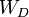$W_D\,$, does$W_B + W_D = 1\,$?

At this point in research, evidently not. Current calculations tend to support the idea that there is only about 30% of the density needed from the total of dark matter ($W_D\,$ = 25%) and visible matter ($W_B\,$ = 5%) to equal a critical density$r_0\,$ meaning that if that is all there is, the universe would eventually stop expanding and collapse. 

### Inflation

Inflation refers to the expansion of the universe, its velocity and whether or not it is slowing or gaining in speed.

The Supernova Acceleration Probe (SNAP) is a space observatory planned for possible construction and launch by 2020. It is designed to measure the expansion of the Universe and determine the nature of Dark Energy which some theoretical models employ to explain accelerating cosmological expansion.

### Dark energy

Dark energy, the energy density of empty space, is also called vacuum energy, zero-point energy and the cosmological constant. Dark energy is a theoretical energy which exerts a negative attraction or opposes the positive attraction of matter and causes the universe to expand.

The two fundamental theories of physics are quantum field theory and general relativity. Since quantum field physics determines energy difference, as a matter of convention, it is not well adapted to study energy density in a vacuum. General relativity, on the other hand, studies the energy density of a vacuum by measuring the curvature of spacetime.

A current line of study is surveying the distribution of galaxies in the cosmos. The distribution pattern is compared to the minuscule temperature fluctuations in the cosmic microwave background. Theoretically acoustic waves moving through the early universe created temperature fluctuations, and the fluctuations are correlated to regions of the universe that had slightly higher and lower densities. These regions of varied density are believed to have influenced how matter eventually clumped together through gravitational influences and thereby formed galaxy clusters. Comparing clumping in the early universe to clumping being observed now may possibly allow researchers to ascertain the role dark energy has played in cosmic evolution.

Some of the most recent studies of dark energy in general relativity convey an extremely small amount, a positive cosmological constant of about 6 × 10-27 kilograms per cubic meter, implying a positive energy density of about 9 × 10-10 joules per cubic meter. This is a very small amount, close to zero.

This corresponds with studies that show the universe is expanding—at an increasing rate. Given that gravity attracts ordinary matter, the dark energy seems to be there in sufficient amounts to counteract the effects of gravity in the universe—at least between galaxy clusters.

Quantum field theory posits that the vacuum of space is filled with virtual particles. At the present stage of the evolution of the universe, the vacuum is less than 10-29 gm/cc. However, quantum field theory calculations gives an unrealistically large amount of density of 2 X 1091 gm/cc while the probable vacuum energy density is at least 120 orders of magnitude smaller implying that there is something else involved that is suppressing the energy density. If there is a vacuum energy density at this point in the development of the cosmos, this implies that there is a small residual vacuum energy density that results in a "cosmological constant." This cosmological constant is a mechanism proposed to resolve the difference between the age of the Universe (9 billion years, abbreviated Gyr, for giga year) and the apparent age of the oldest globular cluster (12-14 Gyr) making the clusters older than the universe which is clearly not possible.

Starobinsky and Guth's inflationary scenario, was developed to provide a solution to the flat and old spacetime model and the horizon problem. To do this, the inflationary scenario requires a vacuum energy density. Starobinsky and Guth's scenario proposes a large vacuum energy that existed very briefly early in the history of the Universe when it was dominated by a vacuum energy density, a density that grew exponentially. If the inflationary period were maintained for a sufficient period of time, the density became very large and the curvature of the Universe became very large. Seen from a human perspective, only the horizon is observable so that a spherical universe would appear flat. This would seem to resolve the the observation that the universe is both old and flat.

Quantum field theory arrives at different values, primarily because it does not take gravity into account. Quantum field theory calculations have posited vacuum energy at infinity, nearly infinite, zero and not determined. Reconciling these varied calculations from general relativity and quantum field theory is an important part of developing a quantum gravity theory.